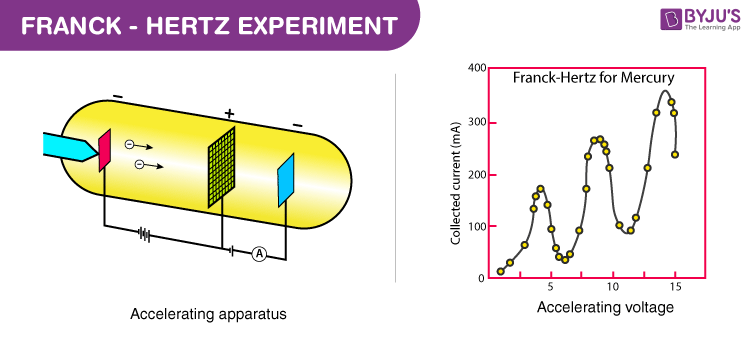# Franck Hertz Experiment

The Franck Hertz experiment was first studied in 1914 by James Franck and Gustav Hertz and presented to the German Physical Society. It was the first electrical measurement to show the quantum nature of atoms. The Franck Hertz experiment consisted of a vacuum tube designed to study the energetic electrons that flew through a thin vapour of mercury atoms. It was discovered that only a specific amount of an atom’s kinetic energy would lose as the electrons collide with the mercury atom.

## Franck Hertz Experiment

### Aim:

To demonstrate the concept of quantisation of the energy levels according to the Bohr’s model of an atom.

### Materials Required:

Following are the list of materials required for this experiment:

• Oven
• A control unit for power supply
• A DC amplifier
• Mercury filled Franck-Hertz tube
• Neon filled Franck-Hertz tube

### Theory:

The original experiment used a heated vacuum tube of temperature 115 °C with a drop of mercury of vapour pressure 100 Pa. Three electrodes, an electron-emitting hot cathode, a metal mesh grid, and an anode are attached to the tube. To draw the emitted electrons, the grid’s voltage is made positive with respect to the cathode. The electric current measured in the experiment results from the movement of electrons from the grid to the anode. The electric potential at the anode is slightly negative than the grid so that the electrons have the kinetic energy same as in the grid. The Franck Hertz experiment was explained in terms of elastic and inelastic collisions between the electrons and the mercury atoms.

The graphs show the dependence of the electric current flowing out of the anode and the electric potential between the grid and the cathode. Following are the observations from the graph:

• With the steady increase in the potential difference, the current increases steadily through the tube.
• The current drops almost to zero at 4.9 volts.
• Again there is an increase in the current as voltage increases to 9.8 volts.
• Again a similar drop is observed at 9.8 volts.Energy absorption from electron collisions in the case of neon gas is seen.. When the accelerated electrons excite the electrons in neon to upper states, they de-excite in such a way as to produce a visible glow in the gas region in which the excitation is taking place. There are about ten peak electrons levels in the range of 18.3 to 19.5 eV. They de-excite by dropping to lower states at 16.57 and 16.79 eV. This energy difference gives the light in the visible range.
Hertz Lenard Observation of light and its photoelectric effect is shown in the video below.## What Is An Elastic Collision?

An elastic collision is defined as an encounter between two bodies such that the total kinetic energy of the two bodies remains the same. During the collision, kinetic energy is first converted to potential energy related to repulsive force between the particles converted back to kinetic energy. Rutherford back-scattering is an example of an elastic collision.

• One-dimensional form of the elastic collision of particles 1 and 2:
 $$\begin{array}{l}\frac{1}{2}m_{1}u_{1}^{2}+\frac{1}{2}m_{2}u_{2}^{2}=\frac{1}{2}m_{1}v_{1}^{2}+\frac{1}{2}m_{2}v_{2}^{2}\end{array}$$

Where,

• m1, m2 are the masses of particles 1 and 2
• u1, u2 are the velocities of particles before the collision
• v1, v2 are the velocities of particles after the collision
• The magnitudes of the velocities of the particles after the collision is given with two-dimensional form:
 $$\begin{array}{l}{v}’_{1}=v_{1}\frac{\sqrt{m_{1}^{2}+m_{2}^{2}+2m_{1}m_{2}cos\Theta }}{m_{1}m_{2}}\end{array}$$ $$\begin{array}{l}{v}’_{2}=v_{1}\frac{2m_{1}}{m_{1}+m_{2}}sin\frac{\Theta }{2}\end{array}$$

Related Articles:

## What Is An Inelastic Collision?

An inelastic collision is defined for the two bodies whose kinetic energies are not conserved due to internal friction. Macroscopic collisions result in effects, vibrations of the atoms and the deformation of the bodies. Following is the formula of one-dimensional collision for particles a and b:

 $$\begin{array}{l}v_{a}=\frac{C_{R}m_{b}(u_{b}-u_{a})+m_{a}u_{a}+m_{b}u_{b}}{m_{a}+m_{b}}\end{array}$$ $$\begin{array}{l}v_{b}=\frac{C_{R}m_{a}(u_{a}-u_{b})+m_{a}u_{a}+m_{b}u_{b}}{m_{a}+m_{b}}\end{array}$$

Where,

• va is the final velocity of the first object after impact
• vb is the final velocity of the second object after impact
• ua is the initial velocity of the first object before impact
• ub is the initial velocity of the second object before impact
• ma is the mass of the first object
• mb is the mass of the second object
• CR is the coefficient of restitution (ratio of final and initial relative velocities)

## Frequently Asked Questions – FAQs

### Who first conducted the Franck Hertz experiment?

In 1914, James Franck and Gustav Hertz performed the Franck Hertz experiment.

### Which experiment explained elastic and inelastic collisions between the electrons and the mercury atoms?

Franck Hertz experiment.

### Franck Hertz experiment supports which model of atom?

This experiment supports the Bohr model of atoms.

### Rutherford back-scattering is an example for which type of collision?

Elastic collision.

### Under which condition bodies undergo inelastic collision?

When the two bodies whose kinetic energies are not conserved due to internal friction undergo inelastic collision.

Hope you have understood in detail about the Franck Hertz experiment. Stay tuned with BYJU’S for more such interesting articles. Also, register to “BYJU’S – The Learning App” for loads of interactive, engaging Physics-related videos and an unlimited academic assist.

Test Your Knowledge On Franck Hertz Experiment!